Chin. Phys. B, 2020, Vol. 29(10): 103701    DOI: 10.1088/1674-1056/aba09f
 ATOMIC AND MOLECULAR PHYSICS Prev   Next

# Quantized vortices in spinor Bose–Einstein condensates with time–space modulated interactions and stability analysis

Yu-Qin Yao(姚玉芹)1,†(), Ji Li(李吉)2
1 Department of Applied Mathematics, China Agricultural University, Beijing 102206, China
2 Department of Physics, Taiyuan Normal University, Taiyuan 030031, China
Abstract

The three-component Gross–Pitaevskii equation with an angular momentum rotational term can be served as a model to study spinor Bose–Einstein condensates (BECs) with time–space modulated interactions. Vortex solutions of the spinor BECs with spatiotemporally modulated interactions are worked out by similarity transformation. Theoretical analysis and numerical simulation of vortex states are demonstrated. Stable vortex states are obtained by adjusting the frequency of the external potential and the spatiotemporally modulated interaction.

Keywords:  spinor Bose-Einstein condensates      spatiotemporal modulation      vortex solution      dynamical stability
Received:  12 May 2020      Revised:  19 June 2020      Published:  05 October 2020
 PACS: 37.25.+k (Atom interferometry techniques) 03.75.Dg (Atom and neutron interferometry)
Corresponding Authors:  Yu-Qin Yao(姚玉芹)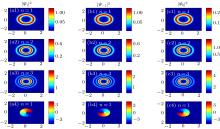Fig. 1.  The density distributions |Ψ± 1,0|2 and phase diagrams at t = 0 of the vortex solution (9) of the rotating spin-1 BECs for topological charge α = 1. (a1)–(a4) Evolution of the density distributions |Ψ1(x,y,0)|2 for different radial quantum numbers n and the phase diagram. (b1)–(b4) Evolution of the density distributions |Ψ–1(x,y,0)|2 for different radial quantum numbers n and the phase diagram. (c1)–(c4) Evolution of the density distributions |Ψ0(x,y,0)|2 for different radial quantum numbers n and the phase diagram. The parameters are taken as Ω = 0.5, μ1 = 8, μ2 = 4, ϵ = 0 and Ω0 = 1.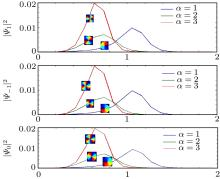Fig. 2.  The density profiles |Ψ± 1,0|2 and phase diagrams at t = 0 for the vortex solution (9) of the rotating spin-1 BECs for different topological charge α and fixed quantum number n = 1. The blue lines denote the density profiles |Ψ± 1,0|2 for the topological charge α = 1, respectively. The green lines denote the density profiles |Ψ± 1,0|2 for the topological charge α = 2 and the red lines denote the density profiles |Ψ± 1,0|2 for the topological charge α = 3, respectively.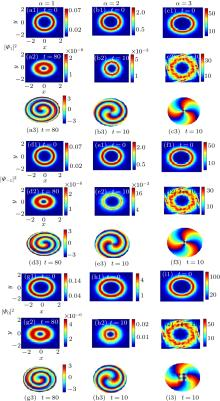Fig. 3.  The evolution of density distributions |ψ± 1,0|2 and phase diagrams for the vortex solution (9) of the rotating spin-1 BECs for different values of topological charge α and fixed quantum number n = 1. The first column is stable vortex for the topological charge α = 1. The second and third columns are unstable vortex for the topological charge α = 2 and α = 3, respectively. The parameters are Ω = 0.5, μ1 = 8, μ2 = 4, ϵ = 0 and ω0 = 1. The domain is (x,y) ∈ [–5,5] × [–5,5] for all the cases.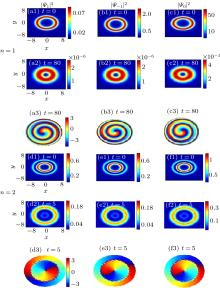Fig. 4.  The evolution of density distributions |ψ± 1,0|2 and phase diagrams for the vortex solution (9) of the rotating spin-1 BECs for the fixed topological charge α and different quantum number n = 1. (a1)–(c3) Evolution of density distributions |ψ± 1,0|2 and phase diagrams for the quantum number n = 1. (d1)–(f3) Evolution of density distributions |ψ± 1,0|2 and phase diagrams for the quantum number n = 2. The parameters are Ω = 0.5, μ1 = 8, μ2 = 4, ϵ = 0 and ω0 = 1.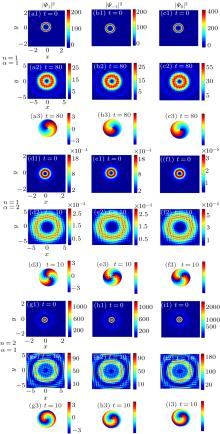Fig. 5.  Time evolution of density distributions |ψ± 1,0|2 and phase diagrams for the vortex solution (9) of the rotating spin-1 BECs for different values of topological charge α and quantum number n. The first three lines show the evolution of density distributions |ψ± 1,0|2 and phase diagrams for n = α = 1. The three lines in the middle show the evolution of density distributions |ψ± 1,0|2 and phase diagrams for n = 1 and α = 2. The last three lines demonstrate the evolution of density distributions |ψ± 1,0|2 and phase diagrams for n = 2 and α = 1. The parameters are Ω = 0.5, μ1 = 8, μ2 = 4, ϵ = 0.1 and ω0 = 0.2.